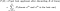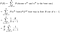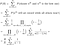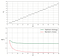# Math-Based Decision Making: The Secretary Problem

## How probability theory answers the question “accept or reject?”

The secretary problem is a famous riddle on the topic of decision making. It is about finding the best strategy when choosing between a sequence of alternatives of which you do not know the best one.

The statement of the secretary problem goes as follows:

You are the HR manager of a company and need to hire the best secretary out of a given number N of candidates. You can interview them one by one, in random order. However, the decision of appointing or rejecting a particular applicant must be taken immediately after the interview. If nobody has been accepted before the end, the last candidate is chosen. What strategy do you use to maximize the chances to hire the best applicant?

At first glance, this problem might appear impossible or seem like a trickery. In reality, the secretary problem has an elegant solution based on sound math.

The practical wisdom coming from the secretary problem is usually lost among the pages of books of probability theory. I think this is a true pity, because there are many situations where knowing the optimal strategy for unseen alternatives can come useful. For example,

• deciding for a flat in a crowded city;

In all these cases, you don’t know what options will come next. However, you might want to decide fast but also appropriately.

The goal of this post is to unveil the solution of the secretaty problem in intelligible words and, only when necessary, a few illustrated steps of math.

You need to make an important decision before proceeding.

You need to decide if you want to read the riddle again and think about the solution on your own. I strongly recommend this, because this is pure brain gymnastics, regardless of you realizing the best strategy or not. If instead you are impatient, then you can also jump straight to the conclusion.

But as Buddha said, “it is better to travel well than to arrive”.

# Randomness? No, Thanks

The secretary problem is challenging because you can’t realize at any point of the interview process if the current applicant is the best one. You can only make comparisons with the candidates interviewed so far. But even when the current candidate has proven to be brilliant, the actual best one could always come right after him.

In front of this complete uncertainty, a tempting strategy is to abandon yourself to luck. You might consider to make an arbitrary decision, such as “Whatever, I choose the first one”. Not surprisingly, this random strategy performs poorly. You only have a probability P=1/N that the first applicant will be the best one. The same is true also when choosing always the last applicant or always the nth one: your odds are always 1/N for any prearranged position.

The random strategy gets worse fast as you increase the number of applicants. If you had just N=3 candidates, choosing at random would only work 33% of the time. With N=10 applicants, the chances of randomly picking the best candidate are a miserable 10%. With N=100, just 1%. These numbers aren’t acceptable for a respectable HR manager.

Is there a viable strategy to outdo randomness?

# Wait. Build Your Benchmark. Wait Again

At this point, you might have realized that the only control variable you have on the entire interview process is rejecting people.

Your strategy can only consist in deciding how many people you want to reject before starting to decide for real. Essentially, you want to wait long enough to get a good reference point and then choose for the next candidate that beats your reference.

1. Interview R people and reject them. Note down who got the highest score. Let’s call this score Xref.

This strategy sounds promising but it is not yet complete: you need to establish the number R of people to reject.

If R is too large, you can build a strong benchmark but you risk to reject the best candidate too. If instead R is too small, you’ll have a dull reference point and you are likely to choose a suboptimal candidate. What we need to do is to find the optimal value R* of people to reject, given N candidates in total. In order to get this, we’ll need some math.

Before starting with math, however, it’s always smart to double-check that the overall idea makes sense. It is convenient to test the considered strategy in the case of N=3 applicants. Here, the only sensical value of people to reject is R=1. Otherwise, you’d always choose the last candidate if R=2, or the first one if R=0, and both cases would be just the random strategy.

The figure below illustrates all the 6 possible arrival combinations of three candidates, together with the respective outcomes of the strategy “reject the first applicant and then pick the next best one (otherwise the last)”.

Our strategy is able to identify the best secretary in three out of the six scenarios. That is, we have a success probability P=1/2. This is definitely better than P=1/3 of the random strategy. Now that our method seems to work, it is worth doing some math.

# Optimizing the Strategy

First, we need to calculate the success probability P(R) of picking the best candidate for some value R of rejected candidates. The success probability can be thought as the sum of the probabilities of finding the best candidate in position n, where n can be comprised between R+1 and the total N (i.e. the remaining candidates):Passage 1: decompose the success probability in mutually exclusive events where the nth applicant is chosen and is also the best. Image by author.

Recall that the accepted candidate is the first one beating the top score of the R rejected candidates. However, this doesn’t guarantee that he is also the best candidate overall.

So, how to calculate the probability that the nth applicant is both chosen and the best one? There are two possible ways.

## Way 1: Reasoning About the 2nd-Best Candidate

The chosen nth candidate is for sure also the best candidate whenever the second-best one was rejected at the beginning. This means that our benchmark was tuned so high to keep rejecting anyone else but the best applicant.

In terms of math, this reasoning translates as follows:Passage 2(a): compute the success probability by imposing that the second-best candidate was discarded among the first R. Image by author.

The last passage just brings the factors independent of n out of the sum. That’s it. We have the formula.

Let’s now consider another insightful way to get to this result.

## Way 2: Reasoning About the Number of Registered Records

The alternative way to require that the nth candidate is both chosen and the best one is to imagine to go sequentially through all of the remaining candidates from R+1 to N and throw a special coin for each.

The coin decides whether that candidate will register a new record score. Due to random ordering, a record happens with probability 1/m, where m is the position of the current candidate. We want only one record outcome for the coin of the nth candidate, whereas all the other coins need to fail a new record with probability 1–1/m.

The mathematical equivalent of this idea readsPassage 2(b): compute the success probability by imposing that a new record score would occur only once up to the last candidate. Image by author.

The last row is just some algebraic manipulation. We verified that the formula is the same as what obtained in the previous way. In math, it is a beautiful moment when two different approaches lead to the same result: it is often a strong hint that you aren’t messing up.

## The Optimal Number of People to Reject

Now that we have a bullet-proof formula for P(R), we can easily compute it numerically for a given number of candidates and see what is the optimal number of rejected people R* that maximizes the success probability P(R).

This can be easily implemented, for instance in the language Julia, as follows:

`Ns = collect(1:50)                       # number of candidatesP(R,N) = R/N*sum([1/(n-1) for n=R+1:N])  # define function P(R)Ropt = zeros(Int64,length(Ns))           # define vector for R*Popt = zeros(length(Ns))                 # define vector for P(R*)for N in Ns                              # optimization loop    Popt[N], Ropt[N] = findmax([P(R,N) for R=1:N])end`

Below, you can see a plot of the optimal R* as N is increased and the corresponding success probability P(R*).(Top) The optimal value of people to reject, as a function of the total number of candidates. (Bottom) In green, the success probability of picking the best candidate following the optimal rejection strategy. In red, the poor success probability of taking a random choice. Image by author.

We can note two interesting trends. First, the success probability with the optimal strategy does not miserably decay towards zero for large N, as for the random choice. This is astounding. With the optimal strategy, the probability of picking exactly the best candidate doesn’t decrease if there are arbitrarily many candidates. Just think about it: it’s more likely to pick the best candidate out of 100 with the optimal strategy than to succeed by choosing at random between 3 applicants.

Secondly, the optimal value R* follows a simple linear relationship with N. Can we extract a practical rule of the thumb out of this? Yes, it is the 1/e Law.

# The 1/e Law

The 1/e Law takes its name from the asymptotic behaviour of the success probability: the ratio 1/e corresponds precisely to the value at which the success probability P(R*) converges for large N. Here, the letter e denotes the Euler’s number, and indeed 1/e is about 1/2.72≈0.37, as can be seen from the plot.

Similarly, we observe that the optimal number of rejected candidates R* grows with N akin to a ladder, by taking a unit jump every 3 or sometimes 2 units. Guess what? The exact slope is again 1/e. This means that the optimal R* can be effectively approximated by computing N/e.

The seemingly magical value 1/e can be explicitly derived by approximating the second last row in the figure “Passage 2(b)” for large R and N, but I’ll omit this computation here. Importantly, the 1/e Law holds true also for more general instances of the secretary problem, for example when also the total number of applicants is uknown but we are given a deadline by which the candidates can apply. For more details about the latter scenario, you can consult this open access paper.

# The Take-Home Message

Do you want to choose at best among a number of take-or-leave alternatives that you don’t know beforehand? Then:

1) reject approximately the first N/2.7 alternatives;

2) choose the next one that outdoes all those seen so far.

I wish you happy decision making!

Special thanks to my dear friend Luca Amato for exposing me to this beautiful math problem.

Written by

Written by

## Lorenzo Duso

#### Physicist passionate about sustainability and applied probability. Track&field freak. PhD Student in Computational Biology.